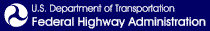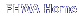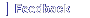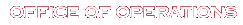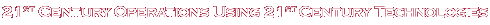# Figure 18. Equations. Objective function and constraints for snowplow routing problem formulation.

## Long Description

Equation 1: min x comma z summation t equals t to t plus T summation k equals one to absolute value of V summation i equals one to absolute value of A summation tao equals t to t plus h summation j equals 1 to n open parenthesis i close parenthesis r open parenthesis s open parenthesis i comma j comma tao close parenthesis close parenthesis q open parenthesis i comma tao close parenthesis where X equals open braces x open parenthesis i comma j comma k comma tao close parenthesis colon for for all i is an element of A comma for all j is an element of open braces one comma ellipsis comma n open parenthesis i close parenthesis close braces comma for all k is an element of V comma tao is an element of open bracket t comma t plus h close bracket close braces and Z equals open braces z open parenthesis i comma k comma tao close parenthesis colon for all i is an element of A comma for all k is an element of V comma for all tao is an element of open bracket t comma t plus h close bracket close braces s period t period.

Equation 2: s open parenthesis i comma j comma tao close parenthesis equals s open parenthesis i comma j comma t close parenthesis plus summation from t prime equals t to tao p subscript t prime open parenthesis one minus summation t prime prime equals t prime minus h underscore p to t prime summation k equals one to absolute value of V x open parenthesis i comma j comma k comma t prime prime close parenthesis close parenthesis.

Equation 3: summation t equals one to T summation i equals one to N x open parenthesis i comma j comma k comma t close parenthesis times the quantity l open parenthesis i close parenthesis divided by v open parenthesis i close parenthesis plus rho summation t equals one to T summation i equals one to N z open parenthesis i comma k comma t close parenthesis plus s t open parenthesis k close parenthesis plus e t open parenthesis k close parenthesis is less than or equal to E T open parenthesis k close parenthesis minus S T open parenthesis k close parenthesis.

Equation 4: x open parenthesis i comma j comma k comma t close parenthesis equals open braces zero otherwise one if j th lane of link i is plowed by vehicle k at time t.

Equation 5: z open parenthesis i comma k comma t close parenthesis equals open braces zero otherwise one if vehicle k has to take a u turn on link i at time t

Equation 6: summation i equals one to N summation j equals one to n open parenthesis i close parenthesis x open parenthesis i comma j comma k comma t close parenthesis is less than or equal to one comma for all i is an element of A comma for all k is an element of V comma for all t is an element of open bracket 0 comma T close bracket.

Equation 7: summation i equals one to N z open parenthesis i comma k comma t close parenthesis is less than or equal to one comma for all i is an element of A comma for all k is an element of V comma for all t is an element of open bracket 0 comma T close bracket.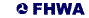United States Department of Transportation - Federal Highway Administration